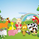Dilihat 3969 kali
Today proposed indicator is based on the corrected moving average, an indicator originally proposed by Andreas Uhl professor at Salzburg University. This moving average is not the most well known, which is a pity since its design is extremely elegant.

The corrected moving average ( CMA ) is an adaptive moving average based on exponential averaging and aim to correct common problems of classical moving averages such as crosses occurring during sideway markets, more details will be introduced in the calculation section. The CMA aim to act as a slow moving average in a moving average crossover system.

Here a new fast adaptive moving average named corrected trend step ( CTS ) based on the CMA is introduced in order to provide a full moving average crossover system based on A. Uhl design.

To Andreas Uhl

Calculation And Understanding The CTS

Even if the code is quite compact, the original idea behind the CMA can be blurry for some users, however it is actually relatively simple to understand. The CMA is based on exponential averaging and a smoothing variable is therefore required, in the CMA the calculation of the smoothing variable is based on the squared distance between the precedent CMA output and a simple moving average , and the rolling variance, where the rolling variance act as threshold.

The CTS work the same way but instead of using the squared error between a simple moving average and the previous CMA output, we use the squared error between the closing price and the previous CTS output, this allow the CTS to better fit with the closing price. As said before the rolling variance act as threshold, if the squared error is lower than the rolling variance this mean that the CTS is close to the price, which can indicate a sideway market, therefore we should filter the entirety of the current price, therefore on sideways market the CTS is equal to the precedent value of the CTS .

In trending/volatile markets we expect the price to go away from the CTS , thus having an high squared error, if the squared error is greater than the rolling variance, the smoothing variable is equal to 1 - variance/squared error, here variance/squared error < 1 since the squared error is greater than the rolling variance (remember that the smoothing variable need to be in a (0,1) range), however if the squared error is way higher than variance this ratio will be small, which would return a non reactive output, but thats not what we want ! This is why we subtract 1 by this ratio in order to make the CTS more reactive instead of less reactive.

In case the squared error is greater than the rolling variance during sideway markets we would not expect a huge difference anyway, that is squared error ≈ variance and therefore:

1 - variance/squared error ≈ 1 - 1/1 ≈ 1 - 1 ≈ 0

This is a beautiful way to make an adaptive moving average , the CMA is not a flashy indicator, but when we look at the details behind the design we can only get amazed, or maybe that its just me, truly a great adaptive moving average .

The System

length control the filtering amount of both moving averages, with higher values of length returning larger filtering amount. Mult multiply the rolling variance by an user selected value, this also allow a greater amount of filtering.

The CTS act as a fast moving average while the CMA act as a slow moving average.

Here the indicator with length = 200, we can see how a sideway market who could have generated a large amount of signals don't affect our system.

Unlike classical crossovers systems where the slow moving average will rarely produce a cross with the fast moving average and price at the same time, the Uhl system can actually do that:

Conclusion

A moving average crossover system based on the corrected moving average proposed by Andreas Uhl has been presented, a new moving average that aim to produce good fits with the price has been created especially for this system. The logic behind the CMA has also been explained. A possible strategy analysis could be presented in the future.

In conclusion i would say the CMA is a bit underrated, in a field where arrows, signals, alerts are the only things appreciated by peoples, original content is slowly dying, this actually make today technical indicators have a pretty bad academic reputations. I'am afraid that today haiku master is Uhl rather than me, i hope to see more indicators from him in the future.

Original paper: http://www.buero-uhl.de/papers.htm
« Je suis las des cruautés de mes semblables, qui ne sont pas mes pareils.

« Je prendrai l’essor et je m’envolerai vers la mer.

« Je connaîtrai le goût des brises du large. J’entendrai les grands cris de la tempête.

## KomenReally interesting chart Alex, I enjoyed studying it. I made an amateur pass at creating a strategy, my hypothesis is that when the CTS line moves the price will want to return back to it. Here's that logic:

strategy.entry("Long", strategy.long, when = cts > cts)
strategy.close("Long", when = close >= cts)

strategy.entry("Short", strategy.short, when = cts < cts)
strategy.close("Short", when = close <= cts)

Seems to eek out some returns, I know there's more to be found in your valuable chart. Thank you again!
BalasHey Alex, that just amazing!